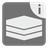Create Intervals (iterator version)
Undefined
English RU

From a set of ints (which will be an iterator of the sorted list) you have to create a list of closed intervals as tuples, so the intervals are covering all the values found in the set. After that you have to create an iterator object which is linked to this list.

A closed interval includes its endpoints! The interval 1..5, for example, includes each value x that satisfies the condition 1 <= x <= 5.

Values can only be in the same interval if the difference between a value and the next smaller value in the set equals one, otherwise a new interval begins. Of course, the start value of an interval is excluded from this rule.
A single value, that doesn’t fit into an existing interval becomes the start- and endpoint of a new interval.

Input: An iterator of the sorted list of ints.

Output: An iterator object linked to the list of tuples.

Examples:

```list(create_intervals(iter(sorted(list({1, 2, 3, 4, 5, 7, 8, 12}))))) == [
(1, 5), (7, 8), (12, 12)]
list(create_intervals(iter(sorted(list({1, 2, 3, 6, 7, 8, 4, 5}))))) == [
(1, 8)]```